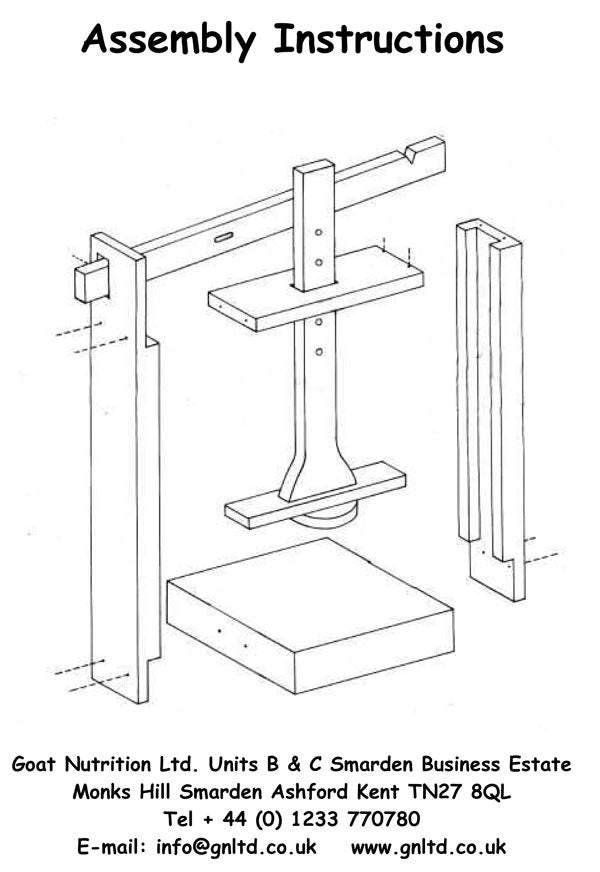# Cheese Press Instructions and Information

Handy info on pressures for small cheese press.

Diameter of piston (i.e. the round wooden bit that pushes down on the cheese) = 78mm (or 3")

Area (π x r²) = 3.14 x 39 x 39 = 4776 sq.mm or approx. 48 sq.cm or 7 sq. ins. (3.14 x 1.5 x 1.5)

The distance from the fulcrum (pivot) to the 'pressure point' (slot in wooden top bar) is approx. 110mm, whereas distance to the weight application point (notch towards the end of top wooden bar) is 330mm, therefore pressure applied at the end gives 330 ÷ 110 which is exactly 3 times the pressure on to the cheese.

So.........if you hang for example a 5kg weight on the notch of the wooden bar and you use the standard round wooden 'piston' to press on the cheese, the pressure on the cheese will be 5kg x 3 = 15kg. This is spread over a 48 sq.cm area which equals 15 ÷ 48=0.31kg/sq.cm or 310g per sq.cm.

If you hang a 5lb weight on the notch the pressure applied would be 3 x 5 = 15lb spread over 7sq.ins = just over 2lbs per square inch.

Please note: for the double cheese press, each wooden piston has an area of 50cm2 (7¾ square inches). The length from the pivot point to the central arm is about 13cm and the length from the central arm to the weight notch is about 22cm, so this gives a pressure ratio of 1.7:1. This means that a 6kg weight hung on the notch gives a pressure of 6 x 1.7 ÷ 50 = 0.204kg/sq.cm or 204g per sq.cm.

If you hang a 10lb weight on the notch the pressure applied would be 1.7 x 10 = 17 lbs spread over 7¾ square inches =  2.2lbs per square inch.